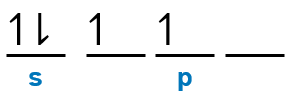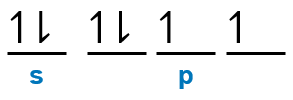# Problem: How many unpaired electrons are present in the ground state of an atom from each of the following groups?1.) Group 4A2.) Group 6A3.) The halogens4.) The alkali metals

###### FREE Expert Solution

All inner electrons are paired, we will look at their valence electrons.

For the orbital diagram, we simply need to draw each orbital and fill them up with the correct number of electrons. Take note that we need to follow Hund’s rule when filling up the orbital diagram. Hund’s rule states that degenerate orbitals (same energy) are first half-filled before being totally-filled.

1.) Group 4A

Group 4A → 4 valence e-

valence electrons: ns2np22 unpaired electrons

2.) Group 6A

Group 6A → 6 valence e-

valence electrons: ns2np42 unpaired electrons

3.) The halogens

82% (437 ratings)###### Problem Details

How many unpaired electrons are present in the ground state of an atom from each of the following groups?

1.) Group 4A

2.) Group 6A

3.) The halogens

4.) The alkali metals